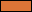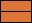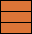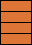Categories

# Balance and Torque Simulation

 Masses 5 kg10 kg15 kg20 kgInstructions : Drag and drop the bricks on both the sides of the balance. Stack them up and see how they balance.

### What is Mass?

It is the measure of how much matter is in an object. The SI unit for mass is Kilogram(kg).

### What is Force?

A force is a push or pull on an object resulting in a change in its motion. The SI unit for force is Newton(N).

### What is a Lever?

A lever is a rigid beam that lies on a point called pivot and is free to rotate about it. It magnifies the amount of force applied due to principle of torque. Levers are very useful simple machines with wide applications.

### What is a Pivot or Fulcrum?

It is the point around which a lever turns. It provides support for the lever.

### What is Torque?

Torque is the measure of a force that can cause an object to rotate about an axis. It is a vector quantity with both magnitude and direction. Amount of torque is given by the magnitude of force multiplied by the distance from the pivot. Torque(Nm) = Force(N) x Distance(m)

### What is Moment?

Moment of force is just another name for torque.